GAN是通过积分概率度量实现数据分布的拟合，从原始的GAN一路发展下来经过了多次重大的改进。Wesserstein距离代替了原始GAN中KullbackLeibler（KL）散度，从而提出了WGAN；梯度惩罚下的WGAN-GP;MMD GAN匹配在希尔伯特空间中单位球上定义的有序矩;SNGAN为代表的参数归一化的实现；Cram’er GAN使用能量距离来训练GAN。这些改进都在对判别器进行约束，本博客将介绍的Sphere GAN使用超球面来绑定目标函数中的积分概率度量（IPM），实现了更加快捷稳定的GAN的训练，同时这篇论文也被选作CVPR 2019 Oral。

# 论文引入

GAN现在已经得到普及式的推广，传统的GAN试图最小化生成数据和真实数据之间的分布差异。为此目的，生成器试图产生看起来像真实数据的所需样本，判别器试图将它们与实际数据区分开。尽管GAN已经成功地应用于各种任务，但是训练它们非常困难，常见的两大问题就是训练上的不稳定（一旦判别器训练到最优状态，生成器会出现参数的梯度消失）、生成的多样性不足（GAN训练上对数据分布的记忆性）。

Sphere GAN是一种新颖的基于IPM的GAN，通过使用几何矩匹配并利用数据的高阶统计信息，从而获得准确的结果。因为矩形匹配是在超球面上进行的，所以Sphere GAN的IPM可以是有界的。文章也证明了超球面引起的几何约束使得GAN训练更加稳定。Sphere GAN并不依赖于传统的基于IPM的GAN的虚拟采样技术和附加的梯度惩罚项，而是利用数学理论支持的黎曼流形下超球面进行实现。

• Sphere GAN是利用黎曼流形在GAN目标函数中定义IPM的第一次尝试
• 第一个基于IPM的GAN下不使用梯度惩罚或虚拟数据采样技术
• Sphere GAN的良好性能在数学上得到证实

# Sphere GAN

$\min_G \max_D \mathbb E_{x \sim \mathcal P}[D(x)] - \mathbb E_{z \sim \mathcal N}[D(G(z))]$

$\min_G \max_D \sum_r \mathbb E_{x \sim \mathcal P}[d_s^r(N,D(x))] - \sum_r \mathbb E_{z \sim \mathcal N}[d_s^r(N,D(G(z)))]$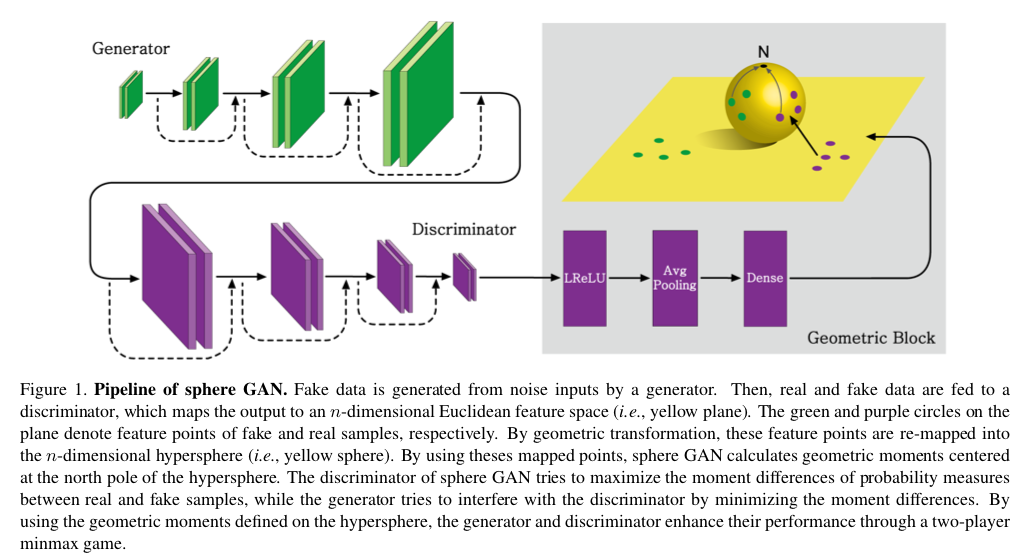• 超球面的距离函数$d_s^r$是有界的
• 梯度范数与$d_s^r$距离函数对于稳定学习至关重要
• 超球面的黎曼结构适用于GAN

# 几何感知转换函数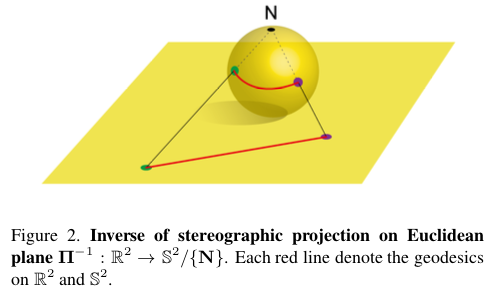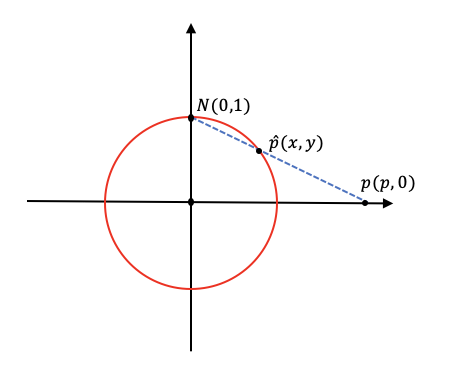$\frac{y}{1} = \frac{p-x}{p}$

$\begin{cases} x = \frac{2p}{p^2+1} \\ y=\frac{p^2-1}{p^2+1} \end{cases}$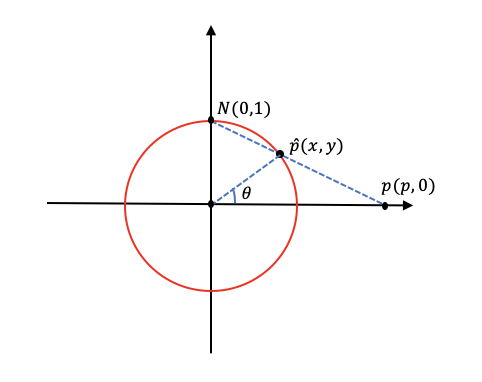$\begin{cases} x = \frac{2p}{p^2+1} = cos \theta \\ y=\frac{p^2-1}{p^2+1} = sin \theta \end{cases}$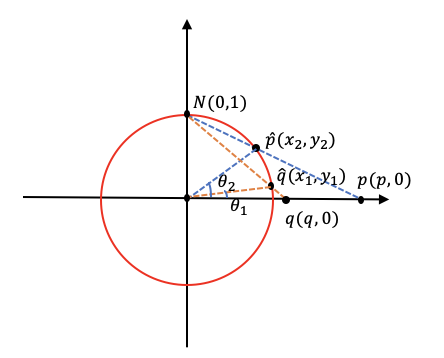$d = arccos(cos(\theta_2 - \theta_1)) \cdot R$

$cos(\theta_2 - \theta_1) = \frac{p^2-1}{p^2+1} \cdot \frac{q^2-1}{q^2+1} + \frac{2q}{q^2+1} \cdot \frac{2p}{p^2+1}$

$d = arccos(\frac{p^2q^2 - p^2 - q^2+ 4pq + 1}{(p^2 + 1)(q^2 + 1)})$

$d_s(\begin{matrix} \prod^{-1} \end{matrix} (p) , \begin{matrix} \prod^{-1} \end{matrix} (q) ) = arccos(\frac{\Vert p^2 \Vert \Vert q^2 \Vert - \Vert p^2 \Vert - \Vert q^2 \Vert + 4pq + 1}{(\Vert p^2 \Vert + 1)(\Vert q^2 \Vert + 1)})$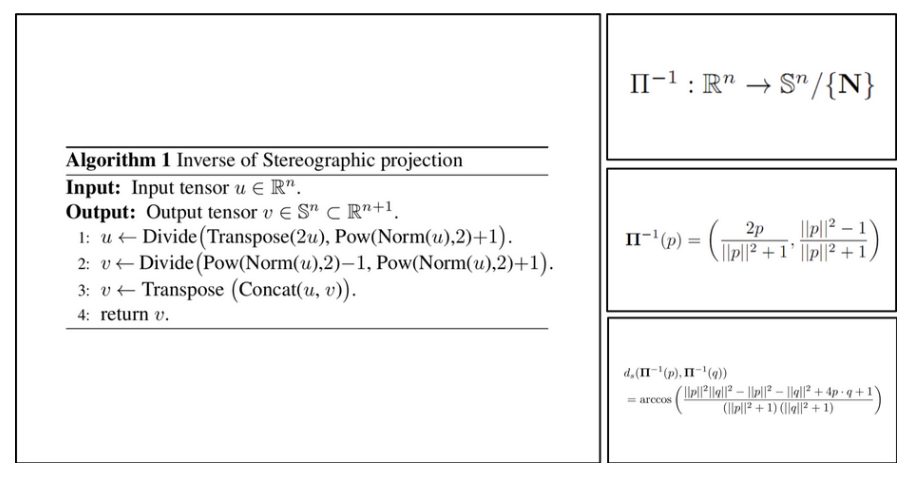def inverse_stereographic_projection(x) :
x_u = tf.transpose(2 * x) / (tf.pow(tf.norm(x, axis=-1), 2) + 1.0)
x_v = (tf.pow(tf.norm(x_u, axis=0, keepdims=True), 2) - 1.0) / (tf.pow(tf.norm(x_u, axis=0, keepdims=True), 2) + 1.0)

x_projection = tf.transpose(tf.concat([x_u, x_v], axis=0))

return x_projection


def sphere_loss(x, y) :
loss = tf.math.acos(tf.keras.backend.batch_dot(x, y, axes=1))
return loss


def discriminator_loss(real, fake, moment=3):
real_loss = 0
fake_loss = 0
bs, c = real.get_shape().as_list()

# [bs, c+1] -> [0, 0, 0, ... , 1]
north_pole = tf.one_hot(tf.tile([c], multiples=[bs]), depth=c+1)
real_projection = inverse_stereographic_projection(real)
fake_projection = inverse_stereographic_projection(fake)

for i in range(1, moment+1) :
real_loss += -tf.reduce_mean(tf.pow(sphere_loss(real_projection, north_pole), i))
fake_loss += tf.reduce_mean(tf.pow(sphere_loss(fake_projection, north_pole), i))

loss = real_loss + fake_loss
return loss

def generator_loss(loss_func, fake, moment=3):
fake_loss = 0
bs, c = fake.get_shape().as_list()

# [bs, c+1] -> [0, 0, 0, ... , 1]
north_pole = tf.one_hot(tf.tile([c], multiples=[bs]), depth=c+1)
fake_projection = inverse_stereographic_projection(fake)

for i in range(1, moment+1) :
fake_loss += -tf.reduce_mean(tf.pow(sphere_loss(fake_projection, north_pole), i))

loss = fake_loss
return loss


# 实验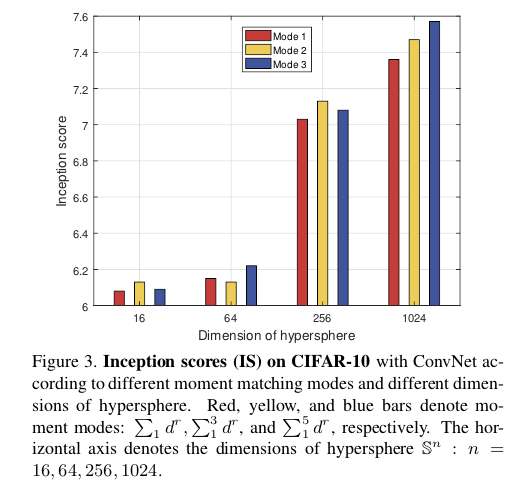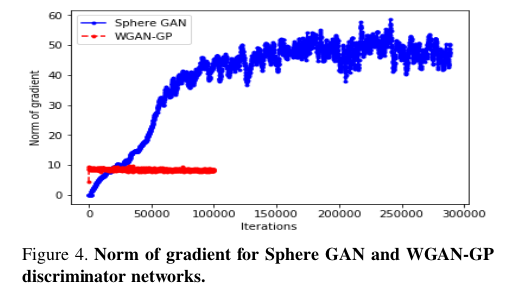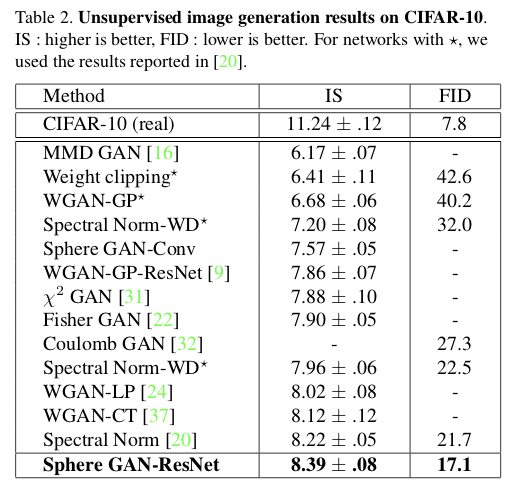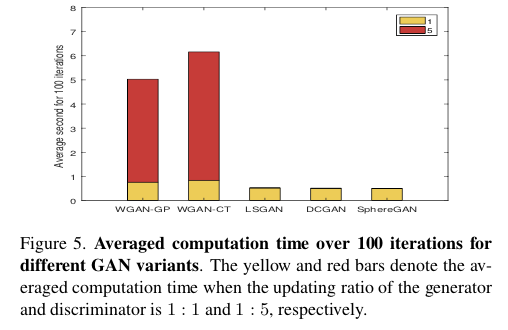# 总结

Sphere GAN是一种新颖的基于IPM的GAN，定义了超球面上的IPM（一种黎曼流形），超球面的黎曼流形提供了有界的IPM使得训练变得稳定。高阶矩匹配使Sphere GAN能够利用有关数据的有用信息并提供准确的结果。实验结果也验证了Sphere GAN确实实现了GAN的又一个不错的方向上的改进。

# 参考文献

 Goodfellow I, Pouget-Abadie J, Mirza M, et al. Generative adversarial nets[C]//Advances in neural information processing systems. 2014: 2672-2680.

 Arjovsky M, Chintala S, Bottou L. Wasserstein generative adversarial networks[C]//International Conference on Machine Learning. 2017: 214-223.

 Gulrajani I, Ahmed F, Arjovsky M, et al. Improved training of wasserstein gans[C]//Advances in Neural Information Processing Systems. 2017: 5767-5777.

 Petzka H, Fischer A, Lukovnicov D. On the regularization of Wasserstein GANs[J]. arXiv preprint arXiv:1709.08894, 2017.

 Wei X, Gong B, Liu Z, et al. Improving the improved training of wasserstein gans: A consistency term and its dual effect[J]. arXiv preprint arXiv:1803.01541, 2018.